# A generalization of multiple zeta values. Part 1: Recurrent sums

Notes on Number Theory and Discrete Mathematics
Print ISSN 1310–5132, Online ISSN 2367–8275
Volume 28, 2022, Number 2, Pages 167—199
DOI: 10.7546/nntdm.2022.28.2.167-199

## Details

### Authors and affiliations

Roudy El HaddadEngineering Department, La Sagesse University
Beirut, Lebanon

### Abstract

Multiple zeta star values have become a central concept in number theory with a wide variety of applications. In this article, we propose a generalization, which we will refer to as recurrent sums, where the reciprocals are replaced by arbitrary sequences. We introduce a toolbox of formulas for the manipulation of such sums. We begin by developing variation formulas that allow the variation of a recurrent sum of order m to be expressed in terms of lower order recurrent sums. We then proceed to derive theorems (which we will call inversion formulas) which show how to interchange the order of summation in a multitude of ways. Later, we introduce a set of new partition identities in order to then prove a reduction theorem which permits the expression of a recurrent sum in terms of a combination of non-recurrent sums. Finally, we use these theorems to derive new results for multiple zeta star values and recurrent sums of powers.

### Keywords

• Recurrent sums
• Partitions
• Multiple zeta star values
• Riemann zeta function
• Bell polynomials
• Stirling numbers
• Bernoulli numbers
• Faulhaber formula

• 11P84
• 11B73
• 11M32
• 05A18

### References

1. Andrews, G. E. (1998). The Theory of Partitions, Vol. 2. Cambridge University Press.
2. Arfken, G. B., & Weber, H. J. (2000). Mathematical Methods for Physicists, 5th Edition. Academic Press.
3. Bernoulli, J. (1689). Propositiones arithmeticae de seriebus infinitis earumque summa finita [Arithmetical propositions about infinite series and their finite sums]. Basel, J. Conrad.
4. Bernoulli, J. (1713). Ars Conjectandi, Opus Posthumum; Accedit Tractatus De Seriebus Infinitis, Et Epistola Gallice scripta De Ludo Pilae Reticularis [Theory of inference, posthumous work. With the Treatise on infinite series… ]. Thurnisii.
5. Bernoulli, J. (1742). Corollary III of de Seriebus Varia. Opera Omnia. Lausanne & Basel: Marc-Michel Bousquet & co. 4:8.
6. Blümlein, J., Broadhurst, D., & Vermaseren, J. A. (2010). The multiple zeta value data mine. Computer Physics Communications, 181(3), 582–625.
7. Blümlein, J., & Kurth, S. (1999). Harmonic sums and Mellin transforms up to two-loop order. Physical Review D, 60(1), 014018.
8. Broadhurst, D. (1986). Exploiting the 1, 440-fold symmetry of the master two-loop diagram. Zeitschrift für Physik C Particles and Fields, 32(2), 249–253.
9. Broadhurst, D. (2013). Multiple zeta values and modular forms in quantum field theory. In Computer Algebra in Quantum Field Theory, Springer, 33–73.
10. Bruinier, J. H., & Ono, K. (2013). Algebraic formulas for the coefficients of half-integral weight harmonic weak Maass forms. Advances in Mathematics, 246, 198–219.
11. Chapman, R. (1999). Evaluating ζ(2). Preprint. Available online at: https://empslocal.ex.ac.uk/people/staff/rjchapma/etc/zeta2.pdf.
12. Euler, L. (1740). De summis serierum reciprocarum [On the sums of series of reciprocals]. Commentarii academiae scientiarum Petropolitanae, 7, 123–134. Opera Omnia, Series, 1, 73–86. Available online at: https://scholarlycommons.pacific.edu/euler-works/41 .
13. Euler, L. (1743). Demonstration de la somme de cette suite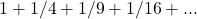[Demonstration of the sum of the series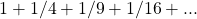]. Journal litteraire d’Allemagne, de Suisse et du Nord, 2, 115–127. Available online at: https://scholarlycommons.pacific.edu/euler-works/63/.
14. Euler, L. (1748). Introductio in Analysin Infinitorum, Volume 1 [Introduction to the Analysis of the Infinite, Volume 1]. Lausanne: Marcum-Michaelem Bousquet, Volume 1, pp. 1–320. Available online at: https://scholarlycommons.pacific.edu/euler-works/101/.
15. Euler, L. (1776). Meditationes circa singulare serierum genus [Meditations about
a singular type of series]. Novi Commentarii academiae scientiarum Petropolitanae, 20, 140–186. Available online at: https://scholarlycommons.pacific.edu/euler-works/477/.
16. Euler, L. (1811). De summatione serierum in hac forma contentarum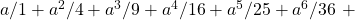etc. [On the summation of series contained in the formetc.]. Memoires de l’académie des sciences de St.-Petersbourg, 3, 26–42. Available online at: https://scholarlycommons.pacific.edu/euler-works/736/.
17. Faulhaber, J. (1631). Academia Algebrae, darinnen die miraculosische Inventiones, zu den höchsten Cossen weiters continuirt und profitiert werden, Ulm, Available online at: https://digital.slub-dresden.de/werkansicht/dlf/254/1.
18. Granville, A. (1997). A decomposition of Riemann’s zeta-function. London Mathematical Society Lecture Note Series, Cambridge University Press, 95–102.
19. El Haddad, R. (2021). Repeated sums and binomial coefficients. Open Journal of Discrete Applied Mathematics, 4(2), 30–47.
20. El Haddad, R. (2022). Repeated integration and explicit formula for the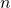-th integral of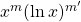. Open Journal of Mathematical Science (in press).
21. El Haddad, R. (2022). A generalization of multiple zeta values. Part 2: Multiple sums. Notes on Number Theory and Discrete Mathematics, 28(2), 200–233.
22. Hardy, G. H., & Ramanujan, S. (1918). Asymptotic formulae in combinatory analysis. Proceedings of the London Mathematical Society, s2-17(1), 75–115.
23. Hoffman, M. E. (1992). Multiple harmonic series. Pacific Journal of Mathematics, 152(2), 275–290.
24. Hoffman, M. E. (1997). The algebra of multiple harmonic series. Journal of Algebra, 194(2), 477–495.
25. Hoffman, M. E., & Moen, C. (1996). Sums of triple harmonic series. Journal of Number Theory, 60(2), 329–331.
26. Kassel, C. (2012). Quantum Groups, Vol. 155. Springer Science & Business Media.
27. Kuba, M., & Panholzer, A. (2019). A note on harmonic number identities, Stirling series and multiple zeta values. International Journal of Number Theory, 15(7), 1323–1348.
28. Loeb, D. E. (1992). A generalization of the Stirling numbers. Discrete Mathematics, 103(3), 259–269.
29. Mengoli, P. (1650). Praefatio [Preface]. Novae quadraturae arithmeticae, seu de additione fractionum [New arithmetic quadrature (i.e., integration), or on the addition of fractions]. Bologna: Giacomo Monti.
30. Murahara, H., & Ono, M. (2019). Yamamoto’s interpolation of finite multiple zeta and zeta-star values. ArXiv. https://doi.org/10.48550/arXiv.1908.09307 .
31. Nielsen, N. (1923). Traité élémentaire des nombres de Bernoulli. Paris, Gauthier-Villars.
32. Oresme, N. (1961). Quaestiones super geometriam Euclidis, Vol. 3. Brill Archive.
33. Propp, J. (1989). Some variants of Ferrers diagrams. Journal of Combinatorial Theory, Series A, 52(1), 98–128.
34. Rademacher, H. (1938). On the partition function p(n). Proceedings of the London Mathematical Society, s2-43(1), 241–254.
35. Schneider, R. (2016). Partition zeta functions. Research in Number Theory, 2(1), 9.
36. Wang, W., & Wang, T. (2009). General identities on Bell polynomials. Computers & Mathematics with Applications, 58(1), 104–118.
37. Xu, C. (2021). Duality Formulas for Arakawa–Kaneko Zeta Values and Related Variants. Bulletin of the Malaysian Mathematical Sciences Society, 44(5), 3001–3018.
38. Zagier, D. (1994). Values of zeta functions and their applications. Proceedings of the First European Congress of Mathematics, Paris, July 6–10, 1992, Springer, 497–512.